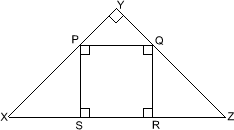#### You may also like### Framed

Seven small rectangular pictures have one inch wide frames. The frames are removed and the pictures are fitted together like a jigsaw to make a rectangle of length 12 inches. Find the dimensions of the pictures.### Tilted Squares

It's easy to work out the areas of most squares that we meet, but what if they were tilted?### Four or Five

The diagram shows a large rectangle composed of 9 smaller rectangles. If each of these rectangles has integer sides, what could the area of the large rectangle be?

# Square in a Triangle

##### Age 11 to 14 Short Challenge Level:

The diagram shows a right-angled isosceles triangle $XYZ$ which circumscribes a square $PQRS$. What is the ratio of the area of square $PQRS$ to the area of the triangle $XYZ$?This problem is taken from the UKMT Mathematical Challenges.
You can find more short problems, arranged by curriculum topic, in our short problems collection.